# Computational Photography--Deconvolution

Posted by GwanSiu on February 13, 2020

## 1. Reasons to Cause Blurry Images

• lens imperfections
• Camera shake
• Scene motioin
• Depth defous

In this post, only blurs caused by lens imperfections and camera shake are discussed.

### 1.1 Lens imperfections

1. What are lens imperfections?
• Ideal lens: A point maps to a point at a certain plane.
• Real lens: A point maps to a circle that has non-zero minimum radius among all plans. The blur kernel is shift-invariant.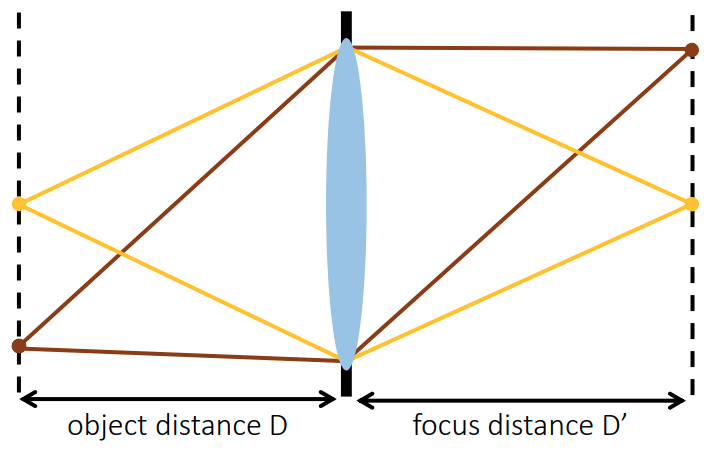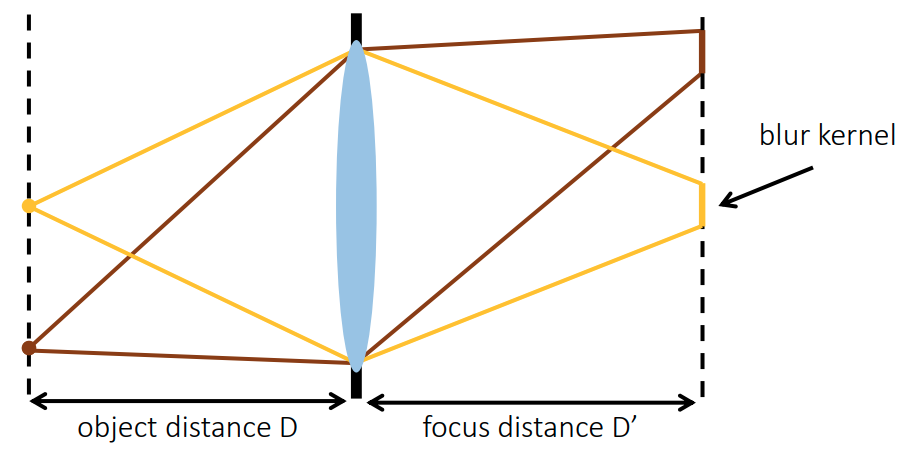1. What causes lens imperfections?
• Aberrations, e.g. Chromatic aberration, spherical aberration, and oblique aberrations. Note: oblique aberrations is not shift-invariant.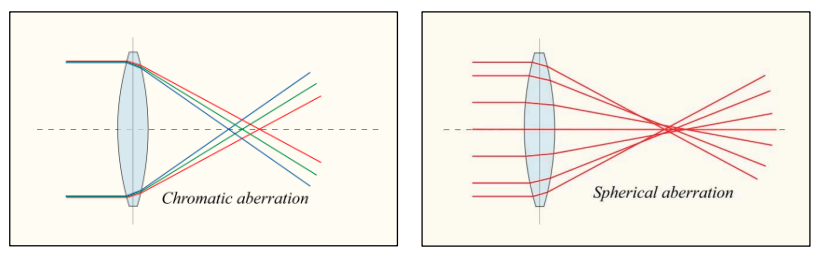• Diffraction, e.g. small aperture, and large aperture.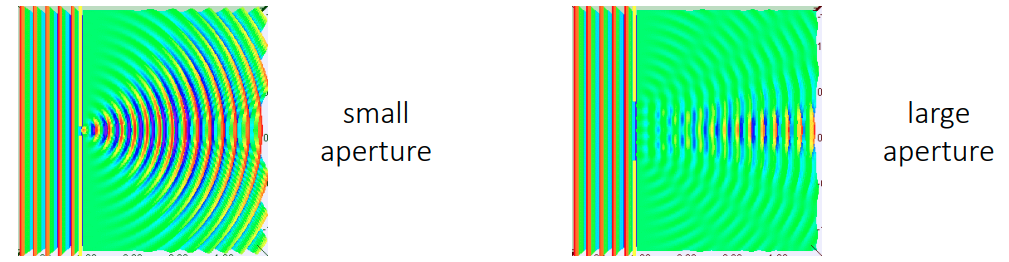1. We condiser lens as a low-pass filter, and we call it point spread function (PSF). The fourier transform of PSF is optical transform function (OTF), which is equatl to aperture shape.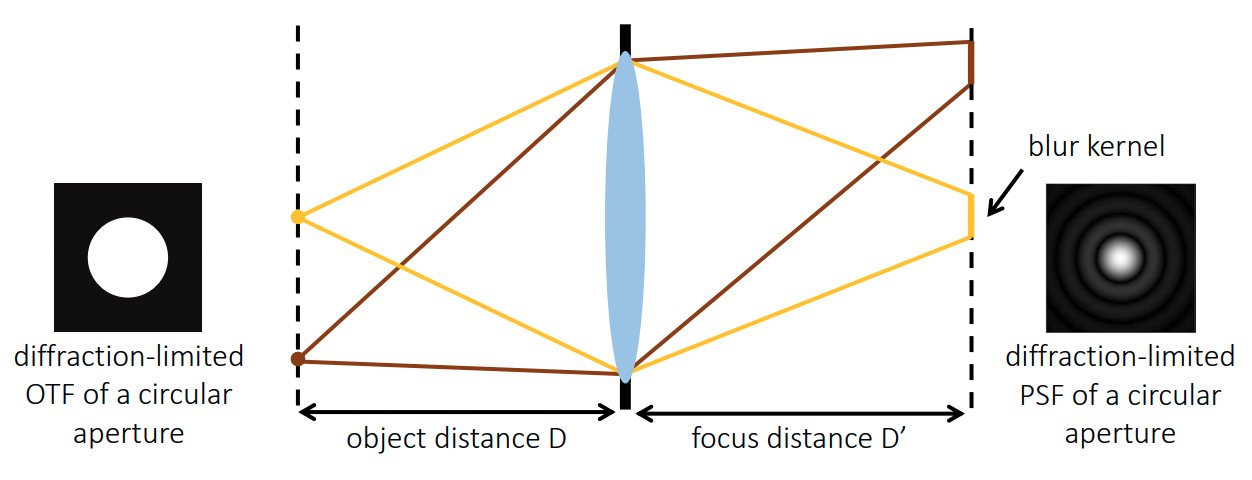## 2. Non-blind Deconvolution

The image sensing model is defined as

where $y$ denotes an observed blurry image, $x$ is the latent image and $b$ represents the blur kernel. $\ast$ denotes the convolution operator.

### 2.1 Inverse Filter

First of all, we consider the smplest case that noise is free in the image sensing model, i.e. $y = x \ast b$. If the kernel information $b$ and the blurry image $bm{y}$ are given, how can we recover the latent image $x$? (Fourier transform)

where $Y=\mathcal{F}(y), X=\mathcal{x}, B=\mathcal{b}$, and $\mathcal{F}(\cdot)$ reprents the Fourier transform. Therefore, we can directly obtain the latent image $x$ by

Limitation: the inverse filter is very sensitive to the noise in the image. In other words, if the observed blurry image contains noise, the inverse filter leads to a poor result.

### 2.2. Winer Filter

In this case, we consider the noise in the image sensing model, i.e. $y = x\ast b + n$. The Fourier transform of the image sensing model is $Y=X\cdot B$.

Problem statements: Find function $H(\omega)$ that minimizes expected error in Fourier domain.

Expand the squares:

Assumption: 1. the latent image and noise are independent. It means that $\mathbb{E}[XN]=\mathbb{X}\mathbb{N}$

1. the expectation of noise is zero, $\mathbb{E}[N] = 0$.

Simplify:

To solve this problem, take it detivative with respect to $H$ and set it to zero. We obtain:

Apply inverse kernel and do not divide by zero

where $1/SNR(\omega)=\frac{\sigma_{s}(\omega)}{\sigma_{n}(\omega)}=\frac{\mathbb{E}[\Arrowvert N\Arrowvert^{2}]}{\mathbb{E}[\Arrowvert X\Arrowvert^{2}]}$.

• derived as solution to maximum-likelihood problem under gaussian noise assumption
• requires estimate of signal-to-noise ratio at each frequency.

Mark:

• 1.Can we undo lens blur by deconvolving a PNG or JPEG image without any preprocessing?
• All the blur processes we discuss are optical degradation
• Blur model is accurate only if our images are linear
1. Are PNG or JPEG images linear?
• No, because of gamma encoding.
• Before deblurring, linearize images first.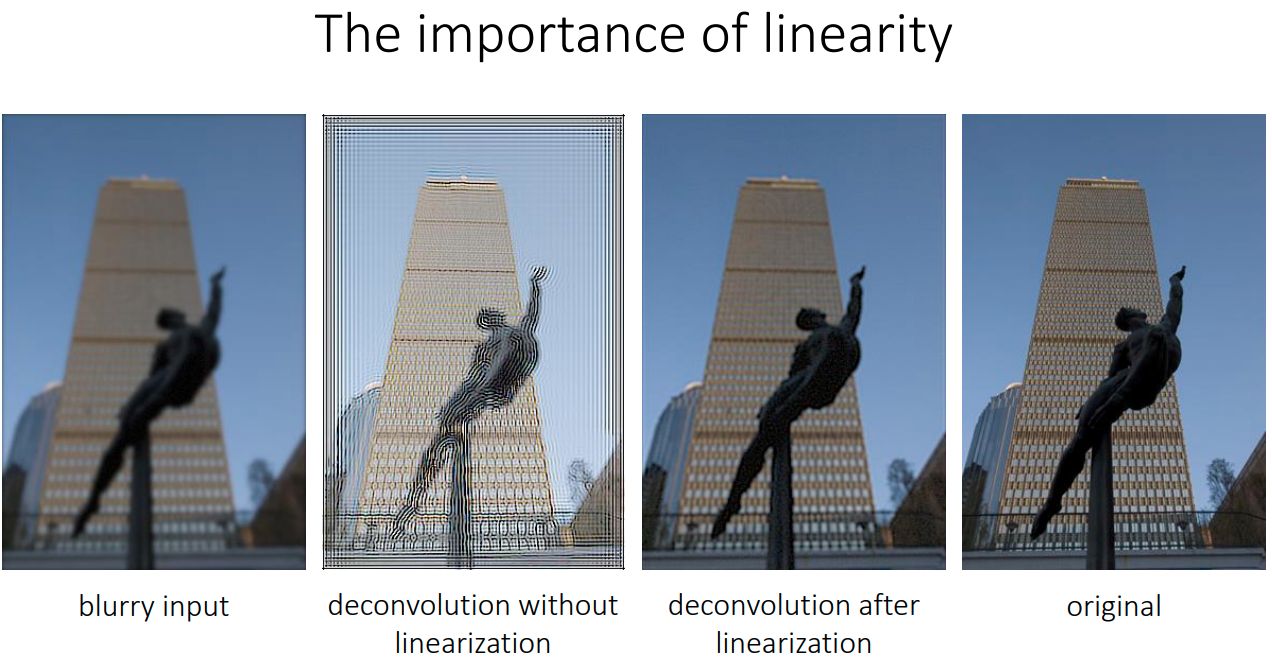1. How do we linearize PNG or JPEG images?
• inverse gamma correction

### 2.3 Generalization Formulation

Such a deconvolution problem that they are ill-conditioned. The optical low-pass filter removes high-frequency information in the original image. Given a PSF or OTF of an optical system, it can be predicted which frequencies are lost. Such an ill-posed problem usually has infinitely solutions, and thus prior information is required, e.g. smoothness, sparseness, sparse gradients, non-local priors, and many others. Without any prior information that is additionally imposed on the estimated solution, it is generally impossible to recover “good” images, which sometimes contains noise and ringing artifacts.

A general formulation for the image reconstruction is

where $x\in\mathbb{R}^{N}$ is a vector of unknown image, $b\in\mathbb{R}^{M}$ is the vectorized measurements, e.g. observed blurry image, and $c\in \mathbb{R}^{N\times M}$ is convolution matrix. Note: the convolution matrix $c$ is a circulant Toeplitz matrix, it means that its eigenvaluess are the Fourier transform of $c$. $\Gamma(x)$ denotes the prior term.

Take an example of the gradient regularization. Generally, for the anisotropic case, the regularizer is modeled as $\Gamma(x)=\lambda\Arrowvert Dx\Arrowvert_{p}$, with $D=[D_{x}^{T}, D_{y}^{T}]^{T}$. $D\in\mathbb{R}^{2M\times N}$ represents the finite differences approximation of the horizontal and vertical image gradients:

where the operator $vec(\cdot)$ vectorize a 2D image and $d_{x}$ and $d_{y}$ are the convolution kernels representing forward finite differences.

• $p=2$, it is $L_{2}$ gradient regularization (Tikhonov regularization).
• $p=1$, it is total variation norm.
• $p=0.8$, it means that gradients of an image are sparsely distributed.

### 2.4 Example

The formulation to the deconvolution problem with TV norm gradient regularization:

we can rewrite the problem formulation as

the augmented Lagragian function is:

where $\mu=(1/ \rho)y$.

#### Efficient implementation of $x$-update

For $x$-update, this is a quadratic program:

we expand it, take it derivative with respect to $x$, and set it as zero. We can get the solution:

$\begin{equation} \bar{x} = (C^{T}C+\rho D^{T}D)^{-1}(C^{T}b+\rho D^{T}v) \end{equation}$ 5)

or using a large-scale, iterative method such as gradient descent, conjugate gradient, or the simultaneous algebraic reconstruction method (SART).

To invert the above equation analytically using inverse filtering, the convolution operation in the spatial domain is converted to the multiplication operation in the frequency domain. We can see that $Cx$ and $Dx$ can be expressed as the convolutions, i.e. $c\ast x$ and $d_{x/y}\ast x$. Therefore, we can quickly solve the problem in the frequency domain:

which gives rise to the inverse filtering proximal operator, which applies only operator and directly obtain the solution:

#### Efficient Implementation of $z$-update

For the $z$-update, this is a LASSO problem, and we can directly get an analytical solution. The the proximal operator is

$\begin{equation} prox_{g,\rho}(v) = \mathcal{S}_{\lambda/\rho}=\arg\min_{z} \lambda\Arrowvert z\Arrowvert_{1} + \frac{\rho}{2}\Arrowvert v - z\Arrowvert_{2}^{2} \end{equation}$ with $v=Dx+\mu$ and $\mathcal{S}_{\kappa}$ being the element-wise soft thresholding operator

that can be implemented very efficiently.

#### The Isotropic case

In isotropic case, the sum of $\ell_{2}$-norms is used to approximate to the horizontal and vertical image gradients as a regularizer. In this case, $z\in\mathbb{R}^{2\times M}$, so that $z=[D_{x}x\, D_{y}y]^{T}$, we can use the $\ell_{2,1}$-norm to write the isotropic version of the regularizer as

this is known as the group lasso problem.

The deconvolution problem with an isotropic TV prior is formulated in ADMM notation as

where $z_{i}$ is the $i$-th element of $z$. For $1\leq i\leq M$, it is meant to represent the finite differences approximattion in horizontal direction, $(D_{x}x){i}$, and for $M+1 \leq i \leq 2M$, the finite differences approximation in vertical direction, $D{y} x_{i}$. Notice that if the $\l_{2}\text{-norm in} g(z)\text{ with the }l_{1}$-norm, then we get $\sum_{i=1}^{M} \Arrowvert(z_{i}, z_{i+M})\Arrowvert_{1}$ which reduces to $\Arrowvert z\Arrowvert_{1}$ and we recover the anisotropic case.

The way to update $x$ and $\mu$ are the same as above, and the only change is the $z$-update, which is

The corresponding proximal operator of $g(z)$, the group lasso, is block soft thresholding. The z-update rule then becomes

$\mathcal{S}_{\kappa}$ being the vector soft thresholding operator.

## 3. Blind Deconvolution

If we only know about the measurement, i.e. observed blurry images $y$, can we recover the unknown image $x$ and kernel $c$?

Ref： Removing camera shake from a single photograph.

Due to the ill-posed nature of the deconvolution problem, prior information plays a significant role when only observed blurry image is given.

• The image “looks like” a natural image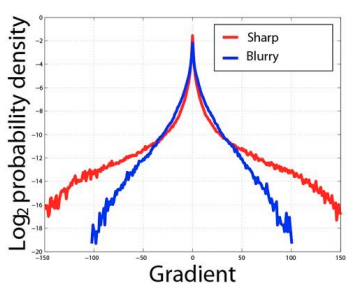• The kernel “look like” a motion PSF.
• Shake kernels are very spars, have continous contours, and are always positive.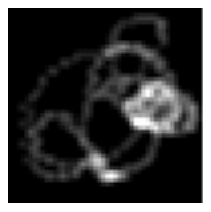### 3.1 Regularized Blind Deconvolution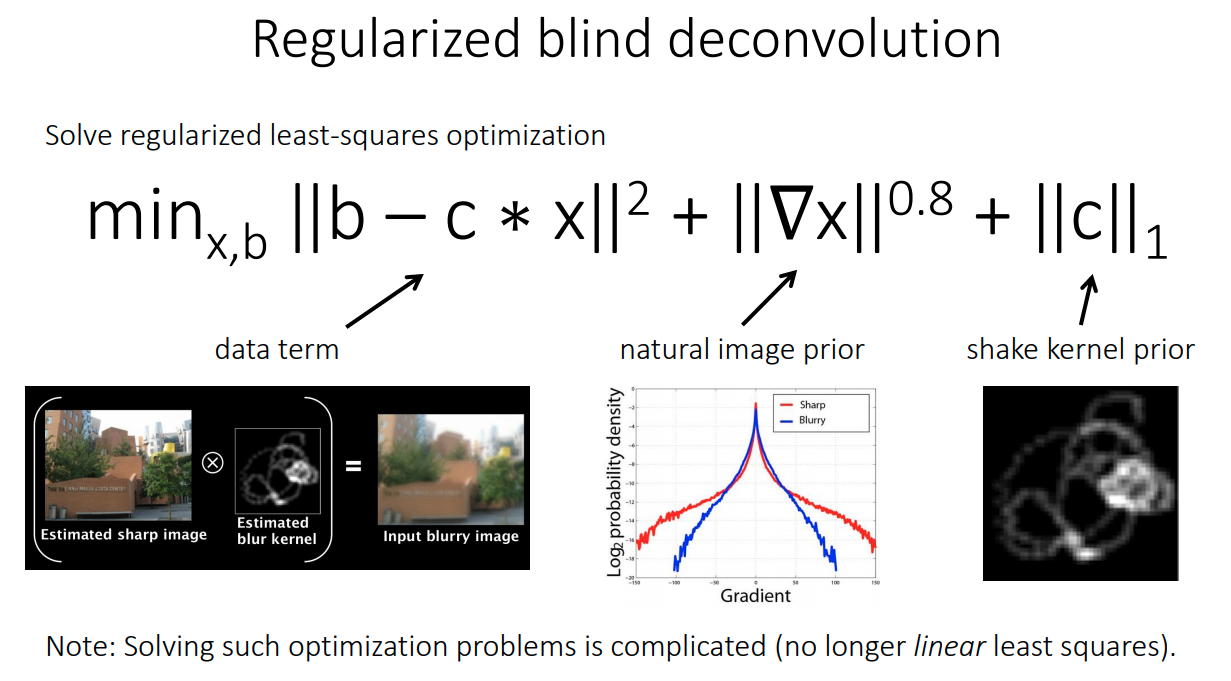## Reference

1. Lecture 12, “Deconvolution”, CMU 12-862, Fall 2018.
2. Lecture 6, “Image Deconvolution”, Stanford EE367/CS4481: Computational imaging and display, Winter 2020
3. Fergus, R., Singh, B., Hertzmann, A., Roweis, S.T. and Freeman, W.T., 2006. Removing camera shake from a single photograph. In ACM SIGGRAPH 2006 Papers (pp. 787-794).